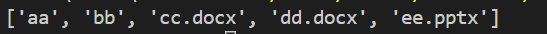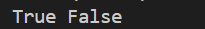python需要读取多个不同深度的文件夹下的某种指定格式的文件,该如何批量操作呢?

• # 一. 常用函数介绍:

## 1. os.listdir()

os.listdir(path)  # 用于返回指定的文件夹包含的文件或文件夹的名字的列表。它不包括 . 和 .. 即使它在文件夹中 。只支持在 Unix, Windows 下使用 。``````import os

dir_path = 'E:/test'
dirs = os.listdir(dir_path)
print(dirs)``````

结果：## 2. os.path.join()

os.path.join(path1, path2, path3,...) # 连接两个或多个路径

``````import os

path1 = 'E:\\'
path2 = 'test'
path3 = 'aa'
dir_path = os.path.join(path1, path2, path3)
print(dir_path)``````

## 3. os.path.isdir()

os.path.isdir(path)  函数判断某一路径是否为目录, 如果是目录, 返回True, 否则返回False。

``````import os

path1 = 'E:\\test\\aa'
path2 = 'E:\\test\cc.docx'
print(os.path.isdir(path1), os.path.isdir(path2))``````

结果：## 4. os.path.isfile()

os.path.isfile(path)  # 判断某一路径是否为文件 ，如果是文件 ，返回True，否则返回False。类似于os.path.isdir()

## 5. os.path.exits()

os.path.exists(path) # 判断路径是否存在, 如果path存在，返回True；如果path不存在 ，返回False 。

``````import os

path1 = 'E:\\test\\aa'
path2 = 'E:\\test\cc'
print(os.path.exists(path1), os.path.exists(path2))``````

## 6. os.mkdir()

os.mkdir(path) # 创建目录，path可以是相对路径或者绝对路径，如果目录有多级 ，则创建最后一级，如果最后一级目录的上级目录有不存在的，则会抛出一个 OSError。

``````import os

path = 'E:\\test\\ff'
os.mkdir(path)``````## 7. copyfile()

copyfile(source_file, distination_file) # 将原内容复制到目标文件中

``````from shutil import copyfile

source_path = 'E:\\test\cc.docx'
destination_path = 'E:\\test\\aa\cc.docx'
copyfile(source_path, destination_path)
``````• # 二. 实例场景及代码:

## 1. 通用代码：

``````import os
from shutil import copyfile
# import sys

def findfile(path):
'''
寻找某一目录下(所有目录深度)的所有某种特定的文件,并对它进行某种操作
'''
lsdir = os.listdir(path)
files = [i for i in lsdir if os.path.isfile(os.path.join(path, i))]
dicts = [i for i in lsdir if os.path.isdir(os.path.join(path, i))]
if files:
for i in files:
if i.split('.')[-1] == 'docx':
# 计数操作,统计所有.docx文件的数目
global num
num+= 1
# 此处可以加任意其他操作
if dicts:
for i in dicts:
findfile(os.path.join(path, i))

num = 0
path = 'G:\wc\PLNet'
findfile(path)
print(num)``````

## 2. 衍生代码：

``````import os
from shutil import copyfile
# import sys

def findfile(path, new_path):
'''
寻找某一目录下(所有目录深度)的所有某种特定的文件,并对它进行拷贝操作
path: 原文件目录
new_path: 拷贝后存放文件的目录
'''
lsdir = os.listdir(path)
files = [i for i in lsdir if os.path.isfile(os.path.join(path, i))]
dicts = [i for i in lsdir if os.path.isdir(os.path.join(path, i))]
if files:
for i in files:
if i == 'raw.lfp':
# 计数操作,统计数目
global num
num+= 1
# 此处可以加任意其他操作,
# 比如将此文件拷贝到某一个指定的目录下,新建每一个文件所在的上一级原目录名,并存放该文件
each_path = os.path.join(new_path, path.split('\\')[-1])
if not os.path.exists(each_path):
os.mkdir(each_path)
copyfile(os.path.join(path, i), os.path.join(each_path, i))
if dicts:
for i in dicts:
findfile(os.path.join(path, i),new_path)

num = 0
path = 'G:\wc\PLNet'
new_path = 'G:\wc\PLNet_new'
if not os.path.exists(new_path):
os.mkdir(new_path)
findfile(path, new_path)
print(num)``````https://blog.csdn.net/qq_28888837/article/details/88060376

https://www.cnblogs.com/sunbeibei/p/13807501.html

https://www.cnblogs.com/keeptg/p/10944109.html